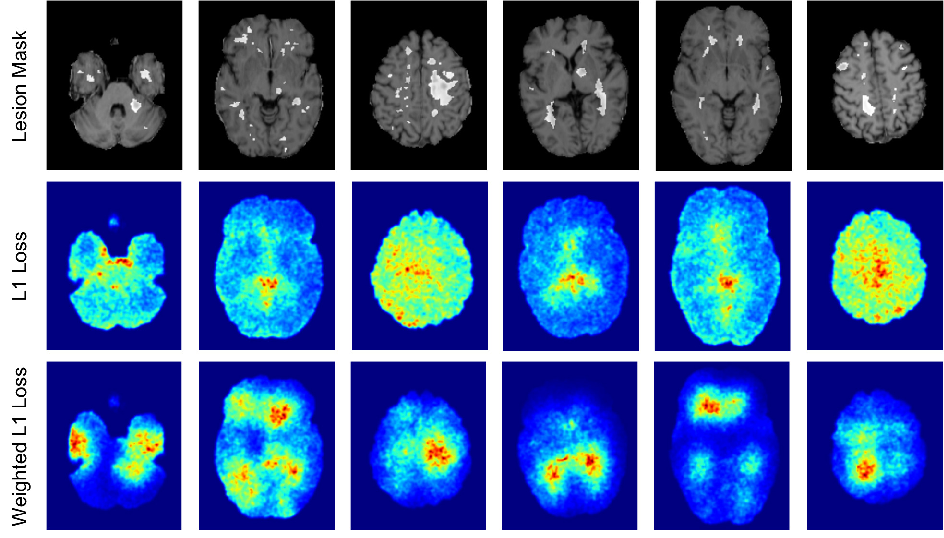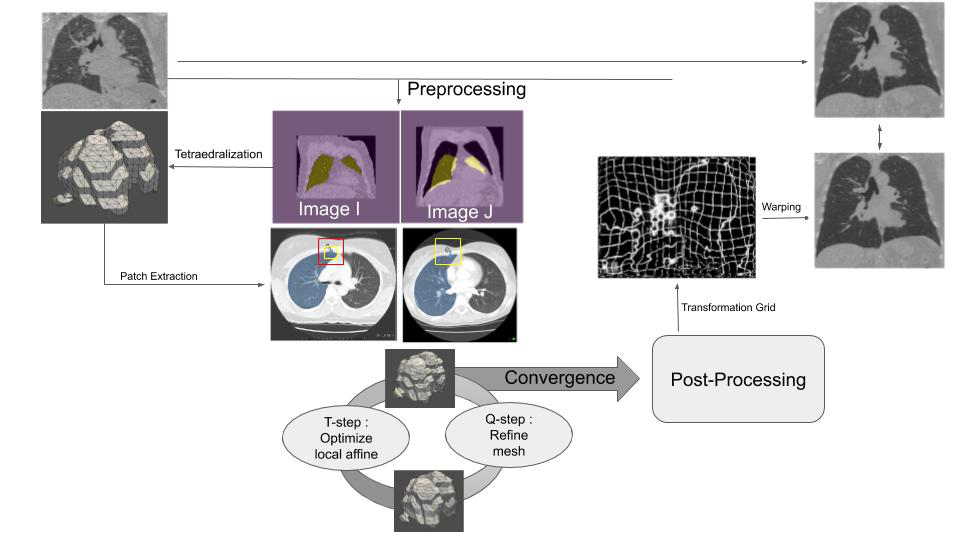EN FR
EN FR

## Section: New Results

### Medical Image Analysis

#### Learning a Probabilistic Model for Diffeomorphic Registration and Motion Modeling

Participants : Julian Krebs [Correspondant] , Hervé Delingette, Tommaso Mansi [Siemens Healthineers, Princeton, NJ, USA] , Nicholas Ayache.

This work is funded by Siemens Healthineers, Princeton, NJ, USA

deformable registration, probabilistic motion modeling, artificial intelligence, latent variable model, deformation transport

We developed a probabilistic approach for multi-scale deformable image registration in 3-D using conditional variational autoencoder ,  and extended it to a motion model by using cardiac MRI image sequences . This includes:

• A probabilistic formulation of the registration problem through unsupervised learning of an encoded deformation model.

• A generative motion model using explicit time-dependent temporal convolutional networks (Fig. 4).

• Demonstration on cardiac cine-MRI for cardiac motion tracking, simulation, transport and temporal super-resolution.

Figure 4. Probabilistic motion model: the encoder ${q}_{\omega }$ projects the image pair (${I}_{0}$, ${I}_{t}$) to a probabilistic low-dimensional deformation encoding ${\stackrel{˜}{z}}_{t}$ from which the temporal convolutional network ${p}_{\gamma }$ constructs the motion matrix $z\in {ℝ}^{d×T}$. The decoder ${p}_{\theta }$ maps the motion matrix to the deformations ${\phi }_{t}$.#### Predicting PET-derived demyelination from multimodal MRI using sketcher-refiner adversarial training for multiple sclerosis

Participants : Wen Wei [Correspondent] , Nicholas Ayache, Olivier Colliot [ARAMIS] .

This work is done in collaboration with the Aramis-Project team of Inria in Paris and the researchers at the Brain and Spinal Cord Institute (ICM) located in Paris.

Multiple Sclerosis, MRI, PET, GANs

By using multiparametric MRI, we proposed to use a 3D FCNN to predict FLAIR MRI which is used clinically for the detection of WM lesions . In addition, we proposed Sketcher-Refiner GANs to predict PET-derived demyelination from multiparametric MRI  with the following contributions:

• Learning the complex relationship between myelin content and multimodal MRI data;

• Comparing quantitatively our approach to other state-of-the-art techniques;

• Proposing visual attention saliency maps to better interpret the neural networks;

• Comparing different combinations of MRI modalities and features to assess which is the optimal input;

Figure 5. The proposed visual attention saliency map. The white regions shown in first row are MS lesion masks. The second row shows some examples of the attention of neural networks when L1 loss is used as the traditional constraint in the loss function, without the specific weighting scheme that we proposed. The third row shows the corresponding attention of neural networks when our proposed weighted L1 loss is applied. It is clear that our designed loss function is able to effectively shift the attention of neural networks towards MS lesions.#### Patch Based Bayesian Mesh Registration

Participants : Paul Blanc-Durand [Correspondant] , Hervé Delingette.

A 1 year grant from APHP

Bayesian Modeling, Mesh deformation, Mechanical model

The objective of this work is to co-register two lung CT scans of the same patient acquired at different breathing cycle based on an elastic and Bayesian model of lung deformation. Its originality stems from the joint estimation of a displacement fields and its derivatives (gradient matrix) defined from a tetrahedral mesh. Inference is performed in two alternating steps including the optimization of local affine transforms and the global optimiztion of the displacement.

Figure 6. Patches are extracted around vertices of mesh. During T-step, we aim to optimize an affine transform centered on a vertice of image I (the moving image) to image J (the fixed image). The affine transform is regularized under a probabilistic model taking into account the deformation of the mesh. During Q-step, we developed an elastical model of lung which homogenize predictions. After few epochs, convergence is achieved.